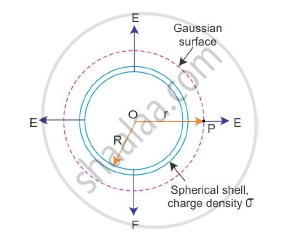# Find the Electric Field Intensity Due to a Uniformly Charged Spherical Shell - Physics

Find the electric field intensity due to a uniformly charged spherical shell at a point (i) outside the shell. Plot the graph of electric field with distance from the centre of the shell.

#### Solution

Let σ be the uniform surface charge density of a thin spherical shell of radius R

Field outside the shell: Consider a point P outside the shell with radius vector r.To calculate E at P, we take the Gaussian surface to be a sphere of radius r with centre O passing through P. All the points on this sphere are equivalent relative to the given charged configuration.

Therefore, the electric field at each point of the Gaussian surface has the same magnitude E and is along the radius vector at each point.

Thus, E and ΔS at every point are parallel, and the flux through each element is E ΔS. Summing over entire ΔS, the flux through the Gaussian surface is E × 4πr2. The charge enclosed is σ × 4πR2.

By Gauss’s law, we get

Exx4pir^2=sigma/epsilon_0xx4piR^2

:.E=(sigmaR^2)/(epsilon_0r^2)=q/(4piepsilon_0r^2)

Where q = 4πR2σ is the total charge on the spherical shell.

The electric field is directed outward if q > 0 and inward if q < 0. This however is exactly the field produced by a charge q placed at the centre O. Thus, for points outside the shell, the field caused by a uniformly charged shell is as if the entire charge of the shell is concentrated at its centre.

Concept: Uniformly Charged Infinite Plane Sheet and Uniformly Charged Thin Spherical Shell (Field Inside and Outside)
Is there an error in this question or solution?
2015-2016 (March) All India Set 3 N

Share